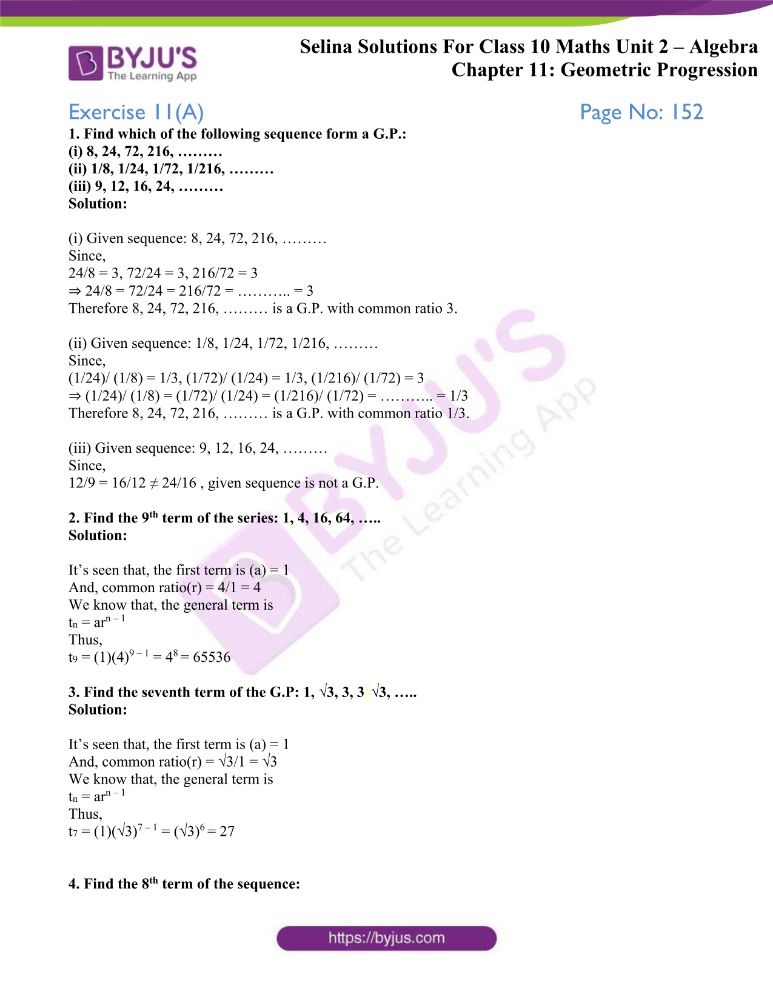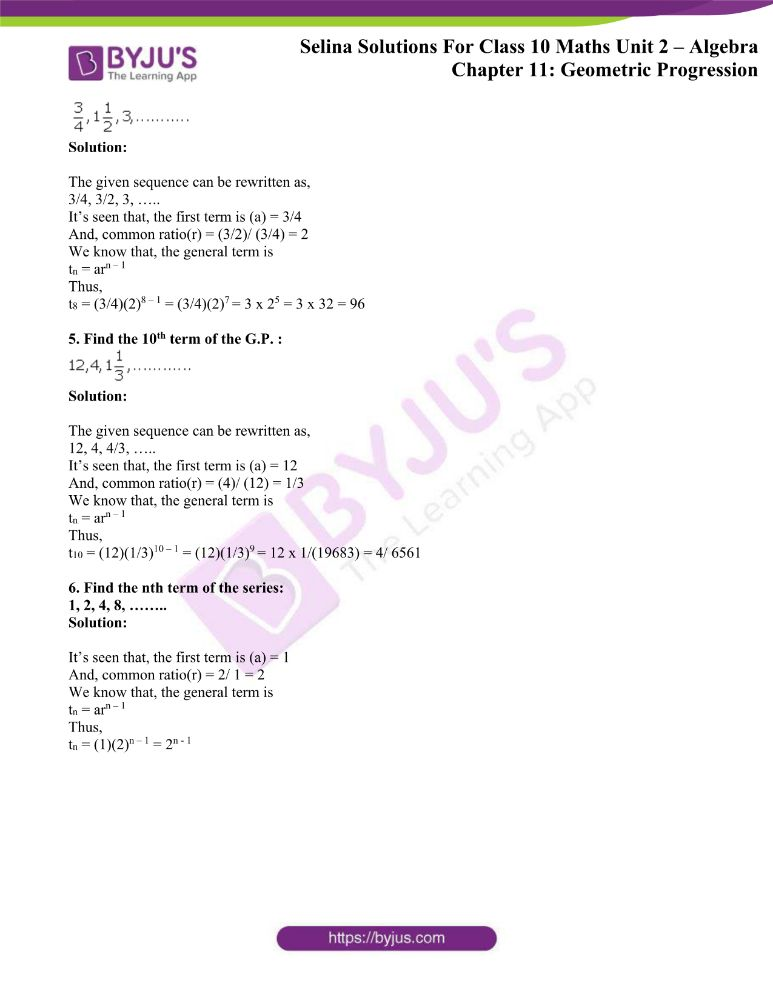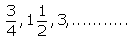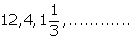# Selina Solutions Concise Maths Class 10 Chapter 11 Geometric Progression Exercise 11(A)

Understanding the general term of a G.P. and finding terms in a G.P. by applying the general term are the primary focus in this exercise. Students wanting to strengthen their basics and get a good grip over the subject can use the Selina Solutions for Class 10 Maths. The solutions are prepared in a simple language to meet the needs of all students. The solutions to the Concise Selina Solutions for Class 10 Maths Chapter 11 Geometric Progression Exercise 11(A) are available in PDF format, provided in the links given below.

## Selina Solutions Concise Maths Class 10 Chapter 11 Geometric Progression Exercise 11(A) Download PDF### Access other exercises of Selina Solutions Concise Maths Class 10 Chapter 11 Geometric Progression

Exercise 11(B) Solutions

Exercise 11(C) Solutions

Exercise 11(D) Solutions

### Access Selina Solutions Concise Maths Class 10 Chapter 11 Geometric Progression Exercise 11(A)

1. Find which of the following sequence form a G.P.:

(i) 8, 24, 72, 216, ………

(ii) 1/8, 1/24, 1/72, 1/216, ………

(iii) 9, 12, 16, 24, ………

Solution:

(i) Given sequence: 8, 24, 72, 216, ………

Since,

24/8 = 3, 72/24 = 3, 216/72 = 3

⇒ 24/8 = 72/24 = 216/72 = ……….. = 3

Therefore 8, 24, 72, 216, ……… is a G.P. with common ratio 3.

(ii) Given sequence: 1/8, 1/24, 1/72, 1/216, ………

Since,

(1/24)/ (1/8) = 1/3, (1/72)/ (1/24) = 1/3, (1/216)/ (1/72) = 3

⇒ (1/24)/ (1/8) = (1/72)/ (1/24) = (1/216)/ (1/72) = ……….. = 1/3

Therefore 8, 24, 72, 216, ……… is a G.P. with common ratio 1/3.

(iii) Given sequence: 9, 12, 16, 24, ………

Since,

12/9 = 16/12 ≠ 24/16 , given sequence is not a G.P.

2. Find the 9th term of the series: 1, 4, 16, 64, …..

Solution:

It’s seen that, the first term is (a) = 1

And, common ratio(r) = 4/1 = 4

We know that, the general term is

tn = arn – 1

Thus,

t9 = (1)(4)9 – 1 = 48 = 65536

3. Find the seventh term of the G.P: 1, √3, 3, 3 √3, …..

Solution:

It’s seen that, the first term is (a) = 1

And, common ratio(r) = √3/1 = √3

We know that, the general term is

tn = arn – 1

Thus,

t7 = (1)(√3)7 – 1 = (√3)6 = 27

4. Find the 8th term of the sequence:Solution:

The given sequence can be rewritten as,

3/4, 3/2, 3, …..

It’s seen that, the first term is (a) = 3/4

And, common ratio(r) = (3/2)/ (3/4) = 2

We know that, the general term is

tn = arn – 1

Thus,

t8 = (3/4)(2)8 – 1 = (3/4)(2)7 = 3 x 25 = 3 x 32 = 96

5. Find the 10th term of the G.P. :Solution:

The given sequence can be rewritten as,

12, 4, 4/3, …..

It’s seen that, the first term is (a) = 12

And, common ratio(r) = (4)/ (12) = 1/3

We know that, the general term is

tn = arn – 1

Thus,

t10 = (12)(1/3)10 – 1 = (12)(1/3)9 = 12 x 1/(19683) = 4/ 6561

6. Find the nth term of the series:

1, 2, 4, 8, ……..

Solution:

It’s seen that, the first term is (a) = 1

And, common ratio(r) = 2/ 1 = 2

We know that, the general term is

tn = arn – 1

Thus,

tn = (1)(2)n – 1 = 2n – 1